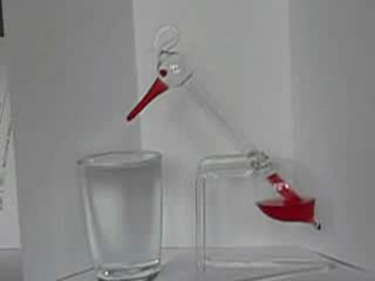# 15.4 Carnot’s perfect heat engine: the second law of thermodynamics

 Page 1 / 7
• Identify a Carnot cycle.
• Calculate maximum theoretical efficiency of a nuclear reactor.
• Explain how dissipative processes affect the ideal Carnot engine.This novelty toy, known as the drinking bird, is an example of Carnot’s engine. It contains methylene chloride (mixed with a dye) in the abdomen, which boils at a very low temperature—about 100ºF . To operate, one gets the bird’s head wet. As the water evaporates, fluid moves up into the head, causing the bird to become top-heavy and dip forward back into the water. This cools down the methylene chloride in the head, and it moves back into the abdomen, causing the bird to become bottom heavy and tip up. Except for a very small input of energy—the original head-wetting—the bird becomes a perpetual motion machine of sorts. (credit: Arabesk.nl, Wikimedia Commons)

We know from the second law of thermodynamics that a heat engine cannot be 100% efficient, since there must always be some heat transfer ${Q}_{\text{c}}$ to the environment, which is often called waste heat. How efficient, then, can a heat engine be? This question was answered at a theoretical level in 1824 by a young French engineer, Sadi Carnot (1796–1832), in his study of the then-emerging heat engine technology crucial to the Industrial Revolution. He devised a theoretical cycle, now called the Carnot cycle    , which is the most efficient cyclical process possible. The second law of thermodynamics can be restated in terms of the Carnot cycle, and so what Carnot actually discovered was this fundamental law. Any heat engine employing the Carnot cycle is called a Carnot engine    .

What is crucial to the Carnot cycle—and, in fact, defines it—is that only reversible processes are used. Irreversible processes involve dissipative factors, such as friction and turbulence. This increases heat transfer ${Q}_{\text{c}}$ to the environment and reduces the efficiency of the engine. Obviously, then, reversible processes are superior.

## Carnot engine

Stated in terms of reversible processes, the second law of thermodynamics    has a third form:

A Carnot engine operating between two given temperatures has the greatest possible efficiency of any heat engine operating between these two temperatures. Furthermore, all engines employing only reversible processes have this same maximum efficiency when operating between the same given temperatures.

[link] shows the $\text{PV}$ diagram for a Carnot cycle. The cycle comprises two isothermal and two adiabatic processes. Recall that both isothermal and adiabatic processes are, in principle, reversible.

Carnot also determined the efficiency of a perfect heat engine—that is, a Carnot engine. It is always true that the efficiency of a cyclical heat engine is given by:

$\text{Eff}=\frac{{Q}_{\text{h}}-{Q}_{\text{c}}}{{Q}_{\text{h}}}=1-\frac{{Q}_{\text{c}}}{{Q}_{\text{h}}}\text{.}$

What Carnot found was that for a perfect heat engine, the ratio ${Q}_{\text{c}}/{Q}_{\text{h}}$ equals the ratio of the absolute temperatures of the heat reservoirs. That is, ${Q}_{\text{c}}/{Q}_{\text{h}}={T}_{\text{c}}/{T}_{\text{h}}$ for a Carnot engine, so that the maximum or Carnot efficiency     ${\mathrm{Eff}}_{\text{C}}$ is given by

${\mathrm{Eff}}_{\text{C}}=1-\frac{{T}_{\text{c}}}{{T}_{\text{h}}}\text{,}$

where ${T}_{\text{h}}$ and ${T}_{\text{c}}$ are in kelvins (or any other absolute temperature scale). No real heat engine can do as well as the Carnot efficiency—an actual efficiency of about 0.7 of this maximum is usually the best that can be accomplished. But the ideal Carnot engine, like the drinking bird above, while a fascinating novelty, has zero power. This makes it unrealistic for any applications.

what does the speedometer of a car measure ?
Car speedometer measures the rate of change of distance per unit time.
Moses
describe how a Michelson interferometer can be used to measure the index of refraction of a gas (including air)
using the law of reflection explain how powder takes the shine off a person's nose. what is the name of the optical effect?
WILLIAM
is higher resolution of microscope using red or blue light?.explain
WILLIAM
can sound wave in air be polarized?
Unlike transverse waves such as electromagnetic waves, longitudinal waves such as sound waves cannot be polarized. ... Since sound waves vibrate along their direction of propagation, they cannot be polarized
Astronomy
A proton moves at 7.50×107m/s perpendicular to a magnetic field. The field causes the proton to travel in a circular path of radius 0.800 m. What is the field strength?
derived dimenionsal formula
what is the difference between mass and weight
assume that a boy was born when his father was eighteen years.if the boy is thirteen years old now, how is his father in
Isru
what is airflow
derivative of first differential equation
why static friction is greater than Kinetic friction
draw magnetic field pattern for two wire carrying current in the same direction
An American traveler in New Zealand carries a transformer to convert New Zealand’s standard 240 V to 120 V so that she can use some small appliances on her trip.
What is the ratio of turns in the primary and secondary coils of her transformer?
nkombo
what is energy
Yusuf
How electric lines and equipotential surface are mutually perpendicular?
The potential difference between any two points on the surface is zero that implies È.Ŕ=0, Where R is the distance between two different points &E= Electric field intensity. From which we have cos þ =0, where þ is the angle between the directions of field and distance line, as E andR are zero. Thus
sorry..E and R are non zero...
By how much leeway (both percentage and mass) would you have in the selection of the mass of the object in the previous problem if you did not wish the new period to be greater than 2.01 s or less than 1.99 s?
hello
Chichi
Hi
Matthew
hello
Sujan
Hi I'm Matthew, and the answer is Lee weighs in mass 0.008kg OR 0.009kg
Matthew
14 year old answers college physics and the crowd goes wild!
Matthew
Hlo

#### Get Jobilize Job Search Mobile App in your pocket Now!ByBy Brooke DelaneyBy Michael SagBy Abby SharpBy Mistry BhaveshBy RhodesBy OpenStaxBy Madison ChristianBy OpenStaxBy Brooke DelaneyBy Steve Gibbs